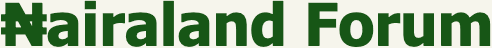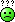#Welcome, Guest: Register On Nairaland / LOGIN! / Trending / Recent / New
Stats: 3,105,837 members, 7,663,863 topics. Date: Friday, 01 December 2023 at 02:20 PM

## How Ethiopia Monk And Scholar Calculate Spring Equinox - Nairaland / General - Nairaland

 How Ethiopia Monk And Scholar Calculate Spring Equinox by Ahari03(m): 6:38pm On Sep 03, 2021 Ethiopian Monks and Scholars traditionally kept 28 tables of 19-year calculations to reckon time in large blocks of 532 years (1.e. 28 x 19 = 532). Those tables contained calculations of calendrical parameters that ultimately allowed the Ethiopians to Calculate Spring Equinox. The scholars made eight sets of calculations to solve this problem and thus compile these calendrical tables.These calculations enabled them to calculate the running total number of differences between the 354-day lunar year and the 365-day solar year. These calculations made extensive use of “modulo” arithmetic. Modulo arithmetic is where there is a limit on the highest number that can be calculated from a procedure.https://iyeyiexprex.com/how-ethiopia-monk-and-scholar-calculate-spring-equinox/Re: How Ethiopia Monk And Scholar Calculate Spring Equinox by Brenn594(m): 11:57pm On Sep 03, 2021 No be dis kontry still dey 2014